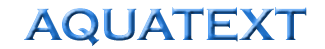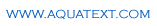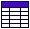The Free On-line Aquaculture DictionaryAmmonia Nitrogen Calculations
 Nitrogen production as a factor of protein fed = 6.25 x protein fed - nitrogen retained in tissues Ammonia produced as a factor of feed fed (assuming commercial diets such as Salmonid) = 0.03 x feed weight % unionised ammonia of total ammonia = 100 / (1 + antilog (pKa -pH)) Where pH = pH value of sample pKa = 0.09018 + 2729.92 / (273 + T) T = Temperature oC
 See also; Ammonia tableand Nitrogen excretion calculations